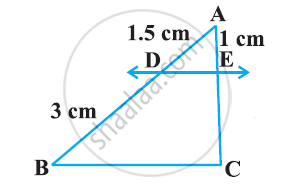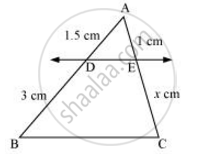# See the given Figure.  DE || BC. Find EC - Mathematics

See the given Figure.  DE || BC. Find EC#### SolutionLet EC = x cm

It is given that DE || BC.

By using basic proportionality theorem, we obtain

(AD)/(DB) = (AE)/(EC)

(1.5)/3 = 1/x

x = (3xx1)/1.5

x =  2

∴ EC = 2 cm

Concept: Similarity of Triangles
Is there an error in this question or solution?

#### APPEARS IN

NCERT Class 10 Maths
Chapter 6 Triangles
Exercise 6.2 | Q 1.1 | Page 128